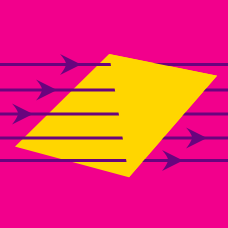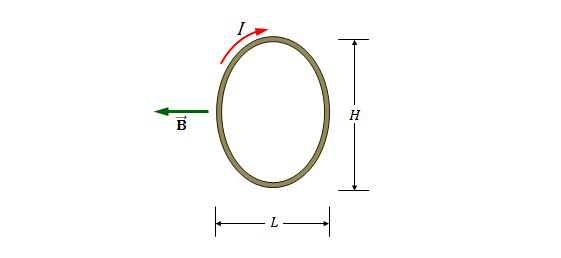Electricity and Magnetism

# Magnetic momentConsider an elliptical shaped single wire loop having a major axis of $H=40.0 \text{ cm}$ and a minor axis of $L=30.0 \text{ cm},$ which is in a uniform magnetic field of $6.00 \times 10^{-4} \text{ T}$ directed toward the left of the screen. The wire loop lies in the plane of the screen and carries a clockwise current of $6.00 \text{ A}.$ What is the approximate magnitude of the torque on the coil?

Note: The area of an ellipse is $A=\pi ab,$ where $a$ and $b$ are, respectively, the semi-major and semi-minor axes of the ellipse.

Suppose that a circular path of radius $r=5.10 \times 10^{-11} \text{ m},$ on which an electron moves with speed $2.40 \times 10^6 \text{ m/s},$ lies in a uniform magnetic field of magnitude $B=7.40 \text{ mT}.$ If we treat the circular path as a current loop, what is the approximate maximum possible magnitude of the torque produced on the loop by the magnetic field?

Assumptions and Details

• The elementary charge is $1.60 \times 10^{-19} \text{ C}.$

Consider a single rectangular coil having dimensions of $3.0 \text{ cm}$ by $7.0 \text{ cm},$ which is placed in a uniform magnetic field of magnitude $8.00 \text{ T}.$ If the maximum torque exerted on it by the magnetic field is $0.25 \text{ N}\cdot\text{m},$ what is the approximate current in the coil?

Suppose that a circular single wire loop of diameter $12.0 \text{ cm}$ is placed in a uniform magnetic field of $4.00 \text{ mT}.$ If the wire loop carries a current of $6.00 \text{ A},$ what is the approximate maximum torque on the wire loop?

Consider a single wire loop in the shape of square made from a copper wire with length $8.0 \text{ m}$ and cross-sectional area $1.2 \times 10^{-4} \text{ m}^2.$ If it is connected to a potential difference of $0.10 \text{ V}$ and then placed in a uniform magnetic field of magnitude $0.50 \text{ T},$ what is the approximate maximum torque that can act on it?

The resistivity of copper is $1.70 \times 10^{-8} \,\Omega \cdot \text{m}.$

×